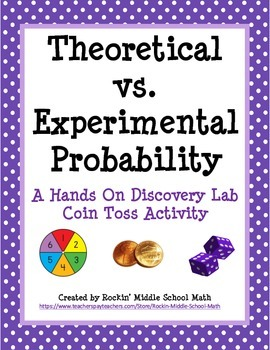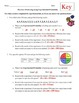Theoretical vs Experimental Probability Lab (includes making predictions)Subject
Resource Type
Common Core Standards
Product Rating
File Type

PDF (Acrobat) Document File

2 MB|6 pages
Share
Product Description

This is a fun, student-centered activity in which students working with a partner will conduct an experimental lab tossing 2 coins. After completing the lab, they will draw conclusions based upon the results of the activity concerning the difference between theoretical and experimental probability. Then they use experimental probability to make predictions. I have included the lab activity, a follow-up worksheet which can be used to engage student discussion after the lab, and a worksheet for further practice as homework, classwork, or an assessment.

This activity is in accordance with the following 7th grade Common Core standards, but may also apply to other grade levels.

CCSS.Math.Content.7.SP.A.2

Use data from a random sample to draw inferences about a population with an unknown characteristic of interest. Generate multiple samples (or simulated samples) of the same size to gauge the variation in estimates or predictions. For example, estimate the mean word length in a book by randomly sampling words from the book; predict the winner of a school election based on randomly sampled survey data. Gauge how far off the estimate or prediction might be.

CCSS.Math.Content.7.SP.C.5

Understand that the probability of a chance event is a number between 0 and 1 that expresses the likelihood of the event occurring. Larger numbers indicate greater likelihood. A probability near 0 indicates an unlikely event, a probability around 1/2 indicates an event that is neither unlikely nor likely, and a probability near 1 indicates a likely event.

CCSS.Math.Content.7.SP.C.6

Approximate the probability of a chance event by collecting data on the chance process that produces it and observing its long-run relative frequency, and predict the approximate relative frequency given the probability. For example, when rolling a number cube 600 times, predict that a 3 or 6 would be rolled roughly 200 times, but probably not exactly 200 times.

CCSS.Math.Content.7.SP.C.7

Develop a probability model and use it to find probabilities of events. Compare probabilities from a model to observed frequencies; if the agreement is not good, explain possible sources of the discrepancy.

Created by Rockin' Middle School Math

https://www.teacherspayteachers.com/Store/Rockin-Middle-School-Math

Total Pages
6 pages